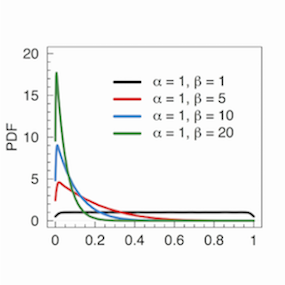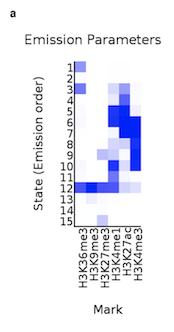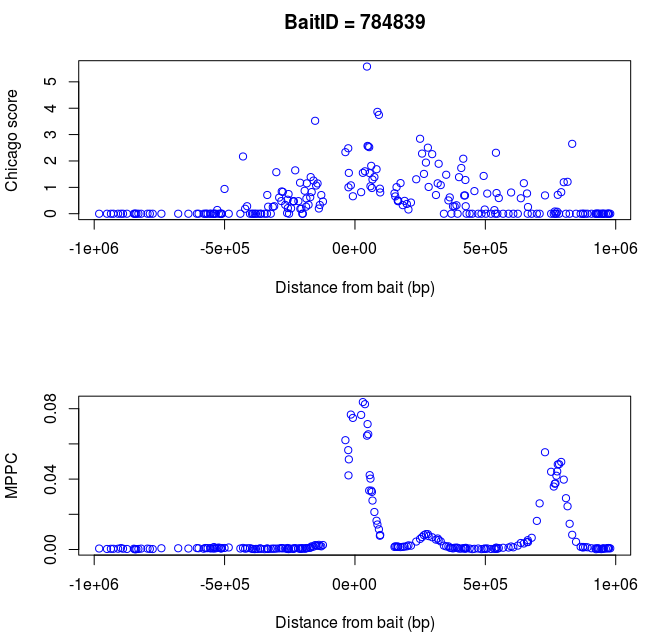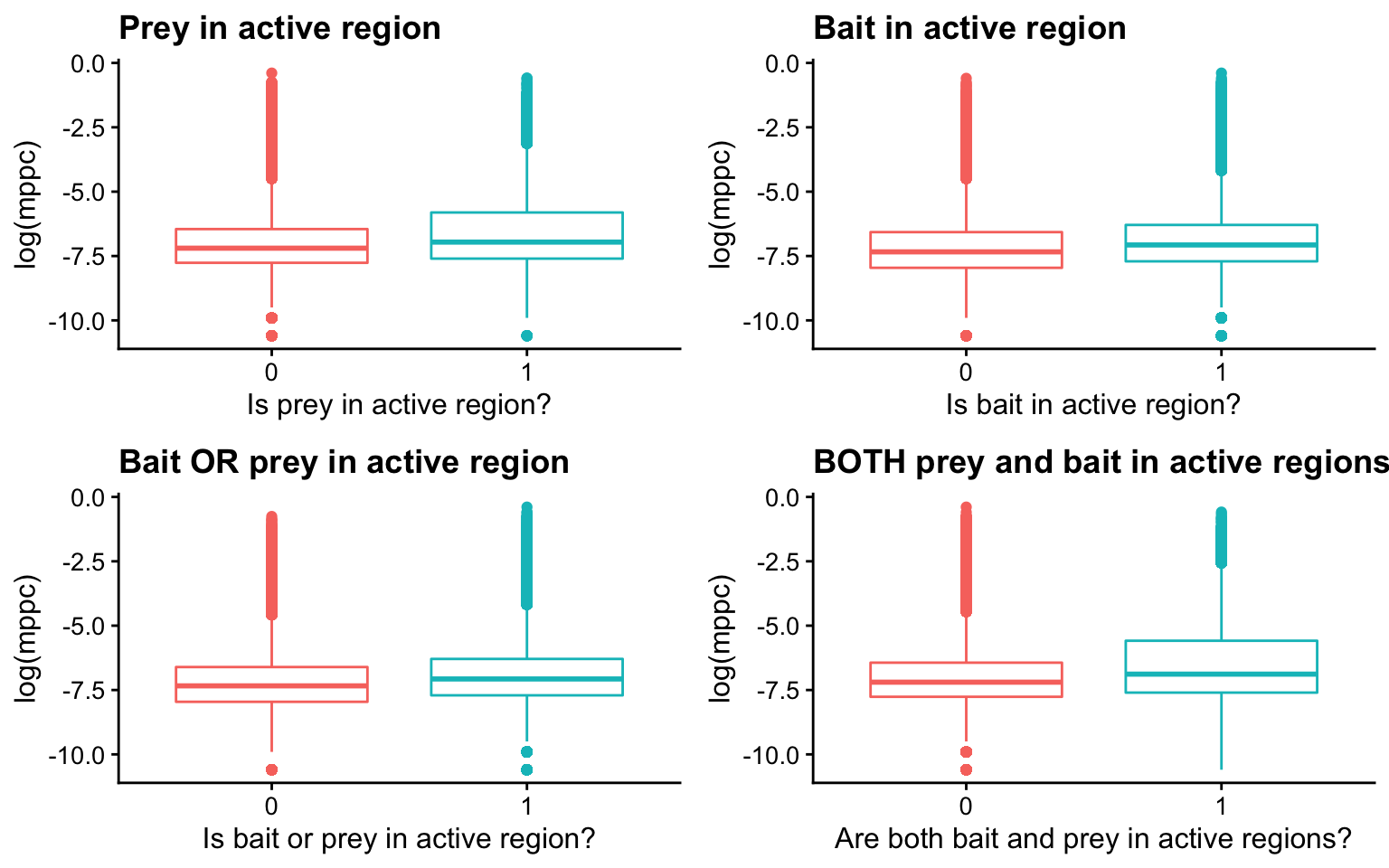### 1. Original peaky model

Peaky jointly models neighbouring prey fragments to fine-map CHi-C interaction data.

The peaky model for a contact between bait, $$b$$, and prey, $$p$$, is

$\begin{equation} Y_{bp}\sim N(\mu_{bp}, 1), \\ \mu_{bp}=\sum_{q:\gamma_{bq}=1}\beta_{bq}\times exp(-\omega d(p,q)). \end{equation}$

Where:

• $$Y_{bp}$$ are the observed NB residuals from the count data

• $$\gamma_{bq}$$ is a binary indicator of a contact between bait $$b$$ and a neighbouring prey fragment $$q$$

• $$\beta_{bq}$$ measures the contact strength between $$b$$ and $$q$$

• $$\omega$$ measures the decay around the location of the bait (currently set to $$10^{-4.7}$$)

• $$d(p,q)$$ is the linear distance between prey $$p$$ and neighbouring prey $$q$$.

So that $$\mu_{bp}$$ measures the expected NB residual.

Peaky uses RJMCMC to sample posterior probabilities for contacts, $$Y_{bp}$$, and contact strengths, $$\beta_{bq}$$. The primary statistic for inference is the marginal posterior probability of a contact (MPPC) between the bait and the prey, which is the proportion of sampled models in which $$\beta_{bq}>0$$.#### Priors

$$\gamma_{bq}$$ is a binary indicator of a contact between bait $$b$$ and fragment $$q$$,

$\begin{equation} \gamma_{bq}\sim Ber(\theta_b). \end{equation}$

We expect few direct contacts and thus encourage sparcity through the specification of a hyper prior on $$\theta_b$$, which is the expected proportion of prey fragments which contact $$b$$. $$F_b$$ is the number of neighbouring prey fragments and so,

$\begin{equation} \theta_b\sim Beta(1, F_b). \end{equation}$

So as the number of neighbouring prey fragments increases, the smaller the probability of a direct contact at $$p$$.

A prior is also placed on the contact strength parameter $$\beta_{bq}$$,

$\begin{equation} \beta_{bq} \sim N(0, \sigma_{\beta}^2),\\ \sigma_{\beta}\sim Unif(0.01, 2) \end{equation}$

In this model, $$\gamma_{bp}$$ and $$\beta_{bp}$$ are inferred in each bait independently of all the other baits.

### 2. Peaky+ ideas

#### 2a. Incorporate external data

We wish to develop the peaky model to incorporate external data on chromatin state into the analysis, so that if there is a chromatin mark indicative of (e.g.) open chromatin at $$q$$, then the the probability of a direct contact between bait $$b$$ and prey $$q$$ increases.

To do this, we could specify another parameter, $$\alpha$$ in the model. So that $$\theta_{bp}$$ is sampled conditional on the external data about chromatin state,

$\begin{equation} \theta_{bp}=\left\{\begin{array}{l}{\theta_0\sim\operatorname{Beta}(1,F_b) \text{, if chromatin inactive}} \\ {\theta_1 \sim \operatorname{Beta}\left(1,\frac{F_b}{\alpha}\right)\text{, if chromatin active}}\end{array}\right. \end{equation}$

where $$\alpha>1$$ and is sampled from some distribution (perhaps gamma), so that the distribution for $$\theta_{1}$$ is more spiked toward 1 (as $$\alpha$$ increases, $$\beta=\frac{F_b}{\alpha}$$ decreases so the expected value increases). To increase information, $$\alpha$$ must be estimated from multiple regions, perhaps even genome-wide.

Note that for now we are assuming that this chromatin state data is binary, but in the future we may like to use continuous data.

#### 2b. Vary $$\omega$$ across baits

The idea above could be extended to sample the decay parameter, $$\omega$$ from some distribution. Note that at the moment, $$\omega$$ is assumed to be fixed and symmetric but this is not biologically sound. For example, if the bait is near the edge of an A/B compartment, then the decay rate would be greater at the side nearest to the boundary as interactions rarely pass over A/B compartment boundaries. For this same reason, the decay would look more symmetric nearer to the centre of an A/B compartment.

Whilst it is likely that $$\omega$$ would also vary within each bait (although the size of baits are much smaller than the size of A/B compartments), this would be very hard and may be subject to over-fitting. Moreover, if we allowed $$\omega$$ to vary within each bait then our prior on the number of peaks within a region becomes very important; for example, is the signal (i) 2 peaks with high decay rate values or (ii) 1 peak with a lower decay rate value. We also can’t use information on A/B compartments to estimate $$\omega$$ because this information comes from the same data, it therefore seems sensible to only allow $$\omega$$ to vary across baits and not within baits (at least for now).

### 3. Characterising active chromatin regionsSide note: Histone modifications associated with transcriptionally active genes and regulatory elements include H3K27ac, H3K4me1 and H3K4me3.

In order to incorporate external information about chromatin state into the analysis, I need to understand how chromatin states (e.g. active/ inactive) are characterised.

CHROMHMM is a tool to help understand the language of epigenetic marks. It uses a hidden Markov model to transition from chromatin marks to chromatin states by integrating multiple tracks from various CHiP-seq data. This is useful because we can jointly visualise the results from various chip-seq experiments (which is also useful because histone modifications are not independent). For example, Burren et al. use CHROMHMM to charactise active/inactive chromatin whereby they input CHiP-seq data for 6 chromatin marks (H3K36me3, H3K9me3, H3K27me3, H3K4me1, H3k27ac, H3K4me3) into a 15 state CHROMHMM model. Each state was then manually annotated and states indicating the presence of promoter or enhancer chromatin tags were selected (E4-E11) to profile regions of active and inactive chromatin. The peaky paper combines these 8 states into a binary measure for active or inactive chromatin, but how is this done?

I can’t find any information about how the continuous data across these 8 states (E4-E11) is converted to a binary value. For now, if any of the columns for E4-E11 states contain a non-zero entry then the binary indicator will be 1 and 0 otherwise. Could also be something to do with the other states, do E1-3 and E12-15 reflect inactive chromatin?

#### Data generation

Using the chromhmm data, I have generated dataframes: final_chrom.RDS, with 5 columns (hindID, chr, start, end and a binary indicator of active or inactive chromatin within this hindiii fragment) and full_chrom.RDS with 13 columns (as final_chrom but with raw emission state values also):

2. Remove any rows where all the emission state values were NA

3. Replace the remaining NAs with 0 (should think of a better way to use this information)

4. Add additional “binary” column which takes a value 1 if any of the state data for states E4-E11 is non-zero and 0 otherwise

5. Load hind-position.RData and use this to add chr, start and end columns representing the genomic positions of the hindiii fragments

The resultant final_chrom.RDS and full_chrom.RDS files have 836,800 rows (original data has 837,157 rows so data for 357 hindiii fragments lost).

The above steps are repeated for the non-activated cells using chromhmm-non.RData instead, creating non_final_chrom.RDS and non_full_chrom.RDS files.

In terms of the peaky data, the baitIDs and preyIDs correspond with the hindIDs (checked by comparing mid.bait column in peaky data and genomic positions of hindIDs in final_chrom). The peaky analysis was ran on four datasets generated from CD4+ T cells; aCD4pchic.RData (activated promoter capture set), aCD4val.RData (activated validation capture set), nCD4pchic.RData (non-activated promoter capture set), nCD4val.RData (non-activated validation capture set). For now, I just focus on the promoter capture datasets.

“Note that the validation set are used here to provide a complementary dataset whose true contacts would be expected to have alternative biological characteristics than the promoter capture set, owing to the opposite fragment being captured.”

I generate dataframes, aCD4pchic_data.RDS and nCD4pchic_data.RDS, with the peaky data and binary columns representing whether the prey, bait, and/or both fall in active chromatin regions.

#### Data analysis

For now, I focus only on the activated T cell dataset.

Example: For bait 784839 the following plot show the chicago scores and the MPPCs. We can see that peaky has adjusted the signals. It seems that there are perhaps 2 direct targets which is not obvious from just looking at the chicago scores. Moreover, peaky expresses it’s uncertaincy (unlike say fine-mapping which just picks out variants) in where exactly these direct targets are. This nice feature comes about because the raw read counts are correlated amongst neighbours and peaky jointly models the decay of the signal around these neighbours directly.Aim: Explore the chromatin state and peaky output data. Start to look at whether PPs from peaky are correlated with chromatin state data –> would expect MPPC to be bigger on average for hindiii fragments falling in regions of active chromatin, is this reflected in the data?

The boxplots shown below are the log transformed mppc values for a random sample of 500,000 rows from the activated CD4 T cell data. We see that when both the bait and prey fall in active chromatin regions, the mppc values are slightly higher (promising).### 4. Quantile Regression

#### Motivation

The prostate cancer paper successfully fine-maps 75 genomic regions using JAM. They use Quantile Regression (QR) to incorporate functional annotation data to prioritise SNPs by regressing the $$PP_{JAM}$$ against a linear combination of annotation features and then updating the posterior probabilities. The motivation for using quantile regression is that these functional annotations (the “prior information”) may help us to differentiate at the extremes of the distribution rather than say the mean, which is what standard regression would tell us.

Here, conditional quantile regression is used to compare statistical and functional evidence for causality. The statistical analysis is performed separate to annotation, as opposed to some fine-mapping frameworks (e.g. CAVIAR) that incorporate functional annotations during the statistical analysis. They justify this by saying that this reduces the potential for strong candidate variants to be downweighted due to experimental artifacts (the annotations come from whole-genome biological data sets so their reliability may be dodgey). The only caveat of this method is the re-use of the data, but this can be overcome by splitting the data into testing and training datasets, and there is enough data (SNPs in this example or peaks in peaky) to do this.

“We believe this more clearly allows the most informative annotations, and the variants that are characterised by those annotations to be highlighted within the data set, whilst also reducing the potential for penalisation of strong candidate variants due to localised artifacts or cell line-specific effects within the whole-genome biological data sets used for annotation. Our conditional QR analysis resulted in adjustment of posterior probability for a small proportion of variants and may further assist prioritisation of the most likely functional variants among the credible set selected for each region.”

To minimise correlations between annotations, single data sets for each transcription factor, histone mark, DNaseI, conserved element and chromatin state by ChromHMM category were selected for investigation.

#### Mathematical method

“At each quantile, we used the fitted model to calculate a predicted posterior probability given the SNP’s annotation features. A single expected posterior probability was then calculated from a weighted average of these quantile-specific expected posterior probabilities with the weight reflecting both the fit (i.e., a function of the likelihood) and variance of the predicted values from the quantile-specific model to the data.”

1. Fit a conditional QR model regressing the PP obtained from JAM ($$PP_{JAM}$$) against the genomic annotation ($$\mathbf{Z}$$) for each quantile $$\tau=(99.2, 99.4, 99.6, 99.8, 99.95\%)$$.

2. Use each of these 5 models to calculate 5 expected PPs for each SNP given the annotation profile for that SNP ($$\hat {PP}_{QR: \tau=99.2}, \hat {PP}_{QR: \tau=99.4}, \hat {PP}_{QR: \tau=99.6}, \hat {PP}_{QR: \tau=99.8}, \hat {PP}_{QR: \tau=99.95}$$).

3. Take a weighted average of these 5 expected PPs for each SNP to yield a final estimate, $$\hat{PP}_{QR}$$.